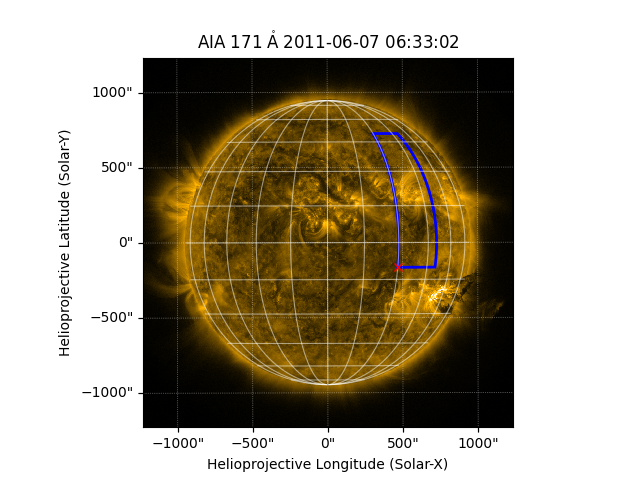How to draw a latitude-longitude quadrangle on a map.

import matplotlib.pyplot as plt

import astropy.units as u
from astropy.coordinates import SkyCoord

import sunpy.map
from sunpy.coordinates import HeliographicStonyhurst
from sunpy.data.sample import AIA_171_IMAGE


The purpose of this example is to demonstrate how to draw a quadrangle on a map using draw_quadrangle(). A quadrangle has edges aligned with lines of constant latitude and longitude in some coordinate system. We start with the sample AIA image.

aia = sunpy.map.Map(AIA_171_IMAGE)


Now let’s define the bottom-left corner of the quadrangle. Note that we define it in a different coordinate frame (heliographic Stonyhurst) than the coordinate frame of the map (which is helioprojective Cartesian).

bottom_left = SkyCoord(30*u.deg, -10*u.deg,
frame=HeliographicStonyhurst, obstime=aia.date)


Now let’s draw a quadrangle on the map, with a width of 20 degrees and a height of 60 degrees. Because the coordinate frame of bottom_left is in HeliographicStonyhurst, the width and height correspond to the longitude and latitude directions, respectively, in that coordinate frame.

fig = plt.figure()